| Technical Review: Pooja Gramopadhye / ABCOM Team | Copy Editor: Anushka Devasthale | Level: Intermediate | Banner Image Source : Internet |
Disclaimer: The purpose of this tutorial is to demonstrate the use of linear regression model on a multi-feature dataset and should not be used as is for predicting admissions.Image source Internet

Are you applying for a Master’s degree program and knowing your chances of admission to your dream university? What GRE score, TOEFL score, or CGPA is required to get an admission in a University of your choice? Learn to apply Linear Regression to develop an ML model to answer these questions.

By the end of this tutorial, you will be able to build and train a linear regression model to predict the chance of admission to a particular university.

# Creating Project

Create a new Google Colab project and rename it to Admit Prediction. If you are new to Colab, then check out this short tutorial.

Import the following libraries in your Colab project:

``````# import statements
import numpy as np
import pandas as pd
import matplotlib.pyplot as plt

from sklearn.model_selection import train_test_split
from sklearn.linear_model import LinearRegression
``````

We will use pandas for data handling, pyplot from matplotlib for charting, sklearn for preparing datasets, and using their predefined machine learning models.

The dataset is taken from Kaggle competition
Use the `read_csv` function of pandas for reading the data file into your Colab project environment.

``````# loading the data from csv file saved at the url
``````

Examine the data by printing the first few records:

``````data.head()
``````

This command gives the following output: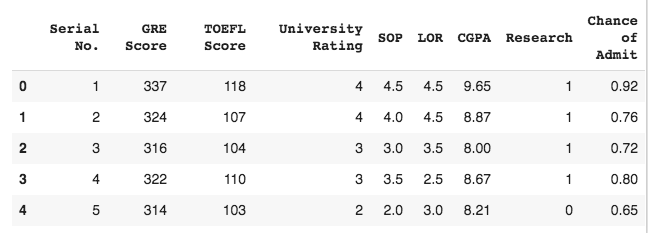As you can see, each row contains the fields such as GRE, TOEFL, SOP, LOR, CGPA scores, and the Research activity of any student along with the university ranking. The last column, Chance of Admit, indicates the chances (probability value) of admission to this school of given ranking. You can check out how many such records are provided in the dataset by calling the `shape` method:

``````# observing the data with the first 5 rows
``````

This command gives an output:
`(500, 9)`
Thus, we have a record of 500 students. We will now proceed to pre-process the data and make it ready for model training.

# Data Pre-processing

We need to ensure that the data does not contain any null values. We do this by calling the `isna` method on the data frame and then taking the sum of values on each column

``````# checking null items
print(data.isna().sum())
``````

This gives the following output:

``````Serial No.           0
GRE Score            0
TOEFL Score          0
University Rating    0
SOP                  0
LOR                  0
CGPA                 0
Research             0
dtype: int64
``````

As all sums are zero, none of the columns have null values. From the above list of columns, you understand easily that Serial No. is of no significance to us in model training. We will drop this column from the dataset:

``````data = data.drop(["Serial No."], axis = 1)
``````

Next, we will prepare our data for building the model.

## Preparing Data

We will first extract the features and the target into two arrays `X` and `y`. You create `X` features array using Python array slicing:

``````X = data.iloc[:,:7]
``````

You can get the information on the extracted data by calling the `info` method. This is the output: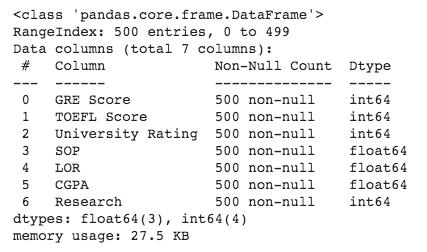As you can see, it contains all our desired features. You now extract target data using the following slicing:

``````y = data.iloc[:,7:]
``````

Print the information on y:

``````y.info()
``````

It shows the following output: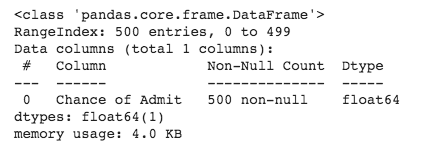We will now split the data into training and testing datasets by calling the `train_test_split` method of sklearn.

``````X_train,X_test,Y_train,Y_test = train_test_split(X, y,
random_state = 10,
shuffle = True,
test_size = 0.2)
``````

I have split the dataset into the ratio 80:20. We use `X_train` and `Y_train` arrays for training and `X_test` and `Y_test` arrays for testing. The training dataset is shuffled to give us the randomness in data. The `random_state` sets the seed for shuffling. Setting the `random_state` ensures reproducible outputs on multiple runs.

We will now get some visualization on the training data so as to decide which model to be used.

## Visualizing Data

We will create charts for each of our features versus the Chance of Admit. This will give us the idea of admission probabilities based on the feature value. For example, how the GRE score affects admission probability? We will be able to get answers to such questions by doing some charting. We first plot the GRE Score feature with the admit probability. We use matplotlib for plotting. The following code produces the desired plot.

``````# Visualize the effect of GRE Score on chance of getting an admit
plt.scatter(X_train["GRE Score"],Y_train, color = "red")
plt.xlabel("GRE Score")
plt.legend(["GRE Score"])
plt.show()
``````

The output is shown below: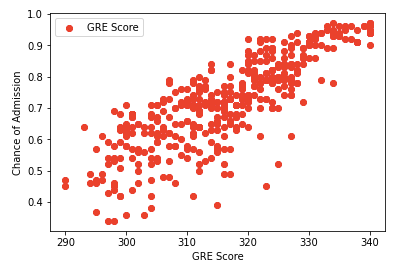You can see that a higher GRE score increases the chances of admission, and the relationship between the two is almost linear.

Now, try plotting a similar graph to see the relation between Chance of Admission and CGPA. You should get the following graph after successfully running the code: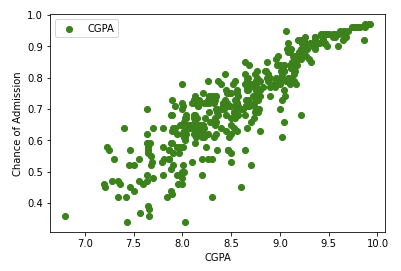Like the first graph, we can see that a higher CGPA has a higher chance of admission, and the relationship is once again linear.

Likewise, try other features and you will see a linear relationship between each of those features, and the admission probability.

Lastly, let us plot the university rating versus the chance of admission.

``````# Visualize the effect of CGPA on chance of of getting an admit.
plt.scatter(X_train["CGPA"],Y_train, color = "green")
plt.xlabel("CGPA")
plt.legend(["CGPA"])
plt.show()
``````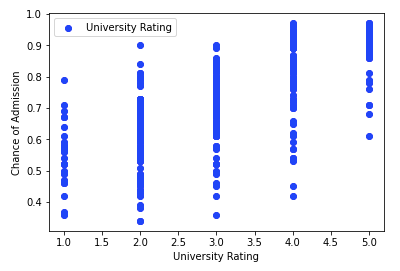In this chart, the relationship is concentrated into five bars. You observe that for university ratings of 2, 3, and 4, the number of admits is the maximum, as decided by the dots' density in those three bars. The admission into universities with rating 1 is low. Similarly, the schools with ratings 5 have a low intake, probably due to their high selection criteria.

We will now build our model.

# Model Building/Training

From the data visualization, we conclude that the relationship between the features and the chances of admission is linear. So, we can try a linear regression model for fitting this dataset.

Our model for this project would be a pre-defined classifier from sklearn library, which is open-source and contains many pre-tested collections of useful classifiers. We will use the LinearRegression from this collection.

``````classifier = LinearRegression()
``````

We call the `fit` method on the classifier to train it. Note the two parameters to the `fit` method.

``````classifier.fit(X_train,Y_train)
``````

The classifier is now ready for testing.

# Testing

To test the classifier, we use the test data generated in the earlier stage. We call the `predict` method on the created object and pass the `X_test` array of the test data, as shown in the following command:

``````prediction_of_Y = classifier.predict(X_test)
``````

This generates a single-dimensional array for the entire testing data set, giving each row prediction in the `X_test` array. Examine the first six entries of this array by using the following command:

``````prediction_of_Y = np.round(prediction_of_Y, decimals = 3)
prediction_of_Y[:6]
``````

The output is: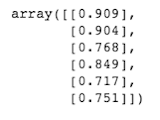If you want to compare the predicted value to the actual value, add the predicted value to `Y_test` and print its contents on screen:

``````Y_test["Predicted chance of Admit"] = prediction_of_Y.tolist()
print(Y_test)
``````

The output is as follows: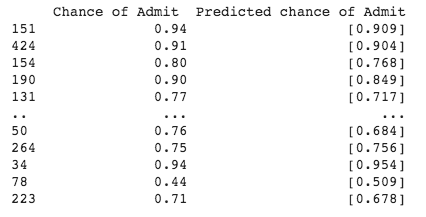As you can see, both the actual and predicted values almost match. We will now drop the added column for further visualizations.

``````Y_test = Y_test.drop(["Predicted chance of Admit"], axis = 1)
``````

But just comparing values on our own is not enough to be sure about the accuracy. We need to verify the accuracy of the prediction.

## Visualizing the predictions

Before verifying the accuracy of the model, we will visualize and compare the difference between the actual chance of admission and predicted chance of admission. This is important because most of the time, we see a model of Linear Regression predicting the result based on only one parameter, and the plot of that is a single line that fits the maximum number of data points. But in this tutorial, we are using multiple parameters, and the graph is complex. So, I have tried to show each parameter's impact on the prediction individually, and I will explain the graphs to make it more evident.

Important things to note before we plot any graphs are plotting two plots in a single graph. The first is of particular parameter against the actual value of Chance of Admit from the testing dataset. The data points of this graph are either red or blue. The second plot is of that same parameter against the predicted value of Chance of Admit. The data points of this graph are purple and red in color.

Let’s plot the first set of graphs for the parameter GRE Score. Use the following code to plot the graphs:

``````# Visualize the difference in graph for same parameter "GRE Score" for actual chance & prediction chance.
plt.scatter(X_test["GRE Score"],Y_test, color = "red")
plt.scatter(X_test["GRE Score"], prediction_of_Y, color='purple')
plt.xlabel("GRE Score")
plt.legend(["Actual chance for GRE Score","Predicted chance for GRE Score"])
plt.show()
``````

Notice that the code contains two calls to scatter function for plotting the two variables.

The output is as follows: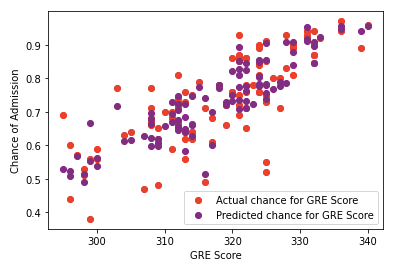Remember that we are plotting the graph from the testing dataset, which contains fewer values than the training dataset. Hence the density of data points in the graph will be less compared to the visualizations on the training dataset. In the above plot, we understand how the GRE Score parameter, which is the same for both plots, produces a different effect for predicted value than the actual value.

Our model's outliers are the red dots at the bottom of the graph because they don’t have any corresponding purple dots around them. How did I infer this from the graph? Considering the error-margin of 5%, a red dot represents a correctly predicted data point if and only if it has a purple dot very near to it, which represents its predicted value. So, the red dots that are isolated are outliers for the model, and the secluded purple dots are poorly predicted values by the model.

This is how you visualize when you are building a Linear Regression model with multiple parameters. The above logic applies to most of the parameters in the model.

Let’s plot another set of graphs for the parameter SOP. Use the following code to plot the graphs:

``````plt.scatter(X_test["SOP"],Y_test, color = "blue")
plt.scatter(X_test["SOP"], prediction_of_Y, color='orange')
plt.xlabel("SOP")
plt.legend(["Actual chance for SOP","Predicted chance for SOP"])
plt.show()
``````

The output is as follows: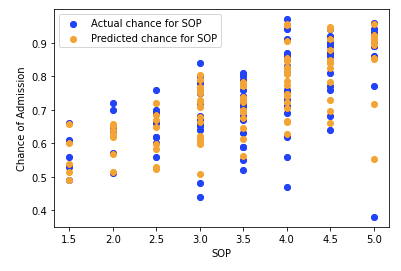Let me explain how to interpret the graph and relate it to the real-world scenarios.

Consider SOP with rating 1.5: The actual chance of admission (blue dots) is near 60%, and predicted chance (orange dots) is near 50%.

Consider SOP with rating 2.5: The actual chance of admission is a lower than the predicted chance.

And this continues for higher SOP as well. Hence this model shows lower chance of admission than an actual for low values of SOP and higher than actual chance for high values of SOP, which is true as SOP is a pivotal factor in getting admission.

Note that these observations are based on the graphs that I have produced with the values of the parameters provided in the tutorial. By changing the values of `shuffle` and `random_state` parameters, all the graphs will also change. You may find some facts if you study your newly produced graphs, and I encourage you to experiment with the code.

Now, we will verify the accuracy of our prediction.

## Verifying Accuracy

To test the accuracy of the model, use the `score` method on the classifier, as shown below:

``````print('Accuracy: {:.2f}'.format(classifier.score(X_test, Y_test)))
``````

The output is:
`Accuracy: 0.80`

It shows that the accuracy of our model is 80%, which is considered good. Thus, no further tuning is required. You can safely try this model with real values to check the chance of getting admission in the desired university. So, now that we know that our model is substantially accurate, we should try the inference on arbitrary values or be more precise real-world values specified by the user.

# Inference on Unseen Data

Let's assume that I have a GRE score of 332, TOEFL score of 107, SOP and LOR of 4.5 and 4.0 respectively, my CGPA is 9.34, but I have not done any research. Let's see what the chances of me getting an admit in a 5.0 rated university are. Use the following code to add all the parameter values in the testing dataset:

``````my_data = X_test.append(pd.Series([332, 107, 5, 4.5, 4.0, 9.34, 0], index = X_test.columns), ignore_index = True)
``````

Check the added row by printing its value:

``````print(my_data[-1:])
``````

Remember that the testing dataset already has some values present in it, and our data will be added in the last row. The following image shows the output of the above code: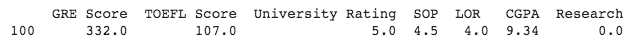Now use the following code to get the chance of admission for the given data:

``````my_chance = classifier.predict(my_data)
my_chance[-1]
``````

The output is as follows:
`array([0.8595167])`

According to our model’s inference, I have an 85.95% chance of getting the admission.

Similarly, you can check admission chances for more than one record as well. Use the following code to add all the parameter values for a bunch of records in the testing dataset:

``````list_of_records = [pd.Series([309, 90, 4, 4, 3.5, 7.14, 0], index = X_test.columns),
pd.Series([300, 99, 3, 3.5, 3.5, 8.09, 0], index = X_test.columns),
pd.Series([304, 108, 4, 4, 3.5, 7.91, 0], index = X_test.columns),
pd.Series([295, 113, 5, 4.5, 4, 8.76, 1], index = X_test.columns)]
user_defined = X_test.append(list_of_records, ignore_index= True)
print(user_defined[-4:])
``````

We use the series data structure of pandas and append all the series to our testing dataset. The code to see the records and predictions is included in the above code. The following image displays the output of the above code: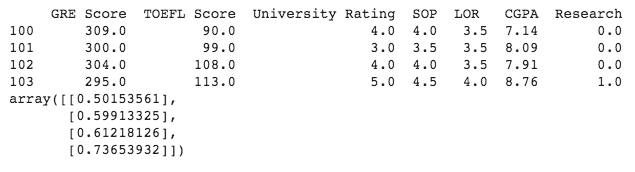Note that the first record is at index 50, and in the previous example with the single record, the index was also 50. This is because when we use the `append` function on data frames, it makes a copy of the original data frame, and changes are made in that copy, leaving the original data frame intact.

By observing the above results, I can assume that CGPA and Research are more important factors than GRE score for getting an admit. Try experimenting with the record values and check the impact it has on the chance of admission. Maybe you will land on a different assumption of your own, or perhaps you will prove me wrong.

Finally, if you just want to do the inference on a single record without adding it to the test dataset, you would use the following code:

``````#Checking chances of single record without appending to previous record
single_record_values = {"GRE Score" : , "TOEFL Score" : , "University Rating" : [4.0], "SOP": [3.5], "LOR" : [4.0], "CGPA": [7.96], "Research": }
single_rec_df = pd.DataFrame(single_record_values, columns = ["GRE Score",  "TOEFL Score",  "University Rating",  "SOP",  "LOR",   "CGPA",  "Research"])
print(single_rec_df)

single_chance = classifier.predict(single_rec_df)
single_chance
``````

This is the output: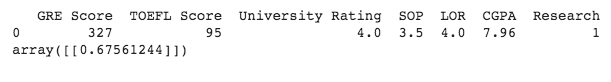Add more values to the list of each parameter in the dictionary to get a chance of multiple records without appending it to `X_test`.

# Summary

In this tutorial, you learned how to develop a linear regression model to create an admission predictor. The first step was selecting an appropriate dataset with all the necessary data needed to build the model. The second step is cleansing the data, eliminating the unwanted rows, fields, and selecting the appropriate fields for your model development. After this was done, you used the `train_test_split` function to map the data into a format that your classifier demands training. For building the model, you used a linear regression classifier provided in the sklearn library. For training the classifier, you used 80% of the data. You used the rest of the data for testing. Then you saw how to visualize the training data by using graphs with the matplotlib library. In the next step, we tested the accuracy of the model. Fortunately, our model had good accuracy. Then you saw how to visualize the results when you are building a Linear Regression model with multiple parameters. Then you saw how to enter user-defined records and predict the chance of admission. This is a very easy model and can be built using many different algorithms, each of which has its pros and cons. Try using some other algorithm for solving this problem.